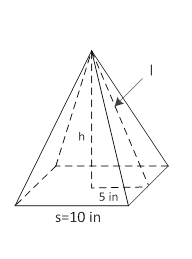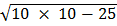### Sample Problem

The base of the following pyramid is a square. If the surface area of the pyramid is 300 in2, what is its volume? Round the answer to the nearest hundredth.V = in3.

#### Solution

SA = Base Area + 4 × Area of a triangle

Area of a triangle = 1/4 × (300 - 10 × 10)

= 50 in2l = (2 × 50) ÷ 10

= 10 in

h == 8.66 in

So, V = 1/3 × 8.66 × 100

= 288.67 in3